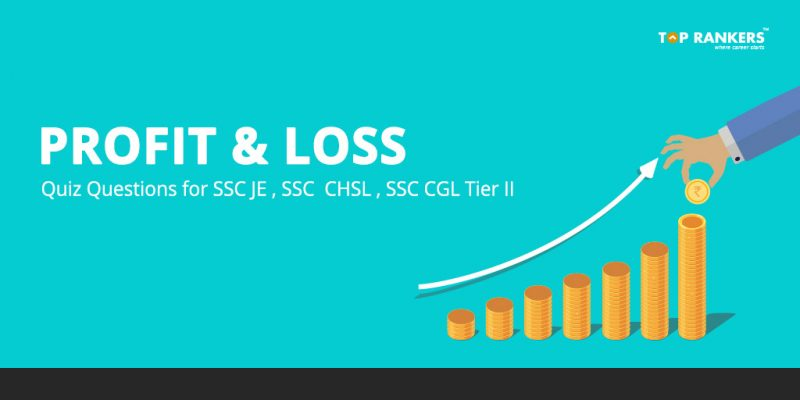# Profit and Loss Quiz Questions for SSC JE , SSC CHSL , SSC CGL Tier IIProfit and Loss Quiz Questions – Get here profit and loss quiz based questions based on latest exam pattern of SSC JE, CHSL, CGL Tier 2

## Profit and Loss Quiz Questions for SSC JE , SSC CHSL , SSC CGL Tier II

If you are preparing for SSC exams, then you are at the right platform. You can practice quiz questions for SSC exams as per the latest exam pattern and syllabus. This quiz is helpful  for various  SSC exams like SSC JE, SSC CHSL and SSC CGL Tier II . Candidates can practice a variety of questions based on profit and loss which will help them to prepare the topic well.

### Profit and Loss Quiz Questions – Tips and Tricks

Profit and Loss is an important part of Numerical Ability/ Quantitative Aptitude section.  You need to be aware of the basic terms and formulas in order to solve questions based on Profit  and Loss.

Some of the important Profit and Loss terms and formulas are given below:

Cost Price (C.P.)

Cost Price is the price at which an article is purchased

Selling Price(S.P.)

Selling Price is the price at which an article is sold,

Marked Price(M.P.)

It is the normal price of an article without any discount.

Profit or Gain

If the Selling Price exceeds the Cost Price, then there is Profit.

Profit = SP – CP

Profit % = Profit/(C P)×100
S P = (100+gain % )/100  ×C P
C P = 100/(100+gain %)×S P

Loss

If the overall Cost Price exceeds the selling price of the buyer then there is a loss.

Loss = C P – S P
Loss % = LOSS/(C P)×100
S P = (100-loss %)/100×C P
C P = 100/(100-loss %)×S P

Take Profit & Loss Quiz

### How to Attempt Profit and Loss Quiz Questions based on SSC JE, CHSL, CGL Tier 2 exam pattern ?

To Attempt our Free Daily Quiz on new topics every day, follow the steps listed below.

1. First of all, click on the direct link given above. Or visit our daily quiz page.
2. Then, register if you haven’t done so already on our website. Existing users can use their credentials to log in to the website.
3. You will be able to see a link ‘Profit and Loss‘. Click on the “Take test” link.
4. Then, follow the instructions and start attempting the quiz.

### Profit and Loss Quiz Question Example:

Example: If a shopkeeper purchased a dozen article for Rs. 360 and sold 7 copies for Rs. 175 then, What is the total Profit/Loss of 8 copies?

Solution:  C.P. of 1 article = 360/12 = Rs. 30
S.P. of a copy= 175/7 = Rs. 25
C.P >S.P. (Loss)
Loss= Cost Price – Selling Price
Loss= 30-25
Loss = Rs. 5

Loss for 8 articles is 5×8 = Rs. 40

### Advantages of taking our Profit and Loss Quiz Questions  for SSC JE, CHSL, CGL Tier 2

• Helps to identify and improve identify weak areas
• Enhances speed
• Improves time-management skills
• Experience a simulated real-exam exam envireonment
• Fun way to learn and improve your performance

### Distinguished Features of Our Quizzes

• Quiz is free to attempt
• Consists of a variety of Questions
• Available in both English and Hindi
• Designed by industry experts

Stay tuned for more Free Daily Quizzes on various topics.Share your thoughts/queries here!